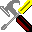#Output Mask

Define the column's print and display format mask. The following mask characters can be used:

 Character Description B Inserts a space character. CR Inserts the "CR" string if the number is negative, or two spaces if the number is positive. DR Inserts the "CR" string if the number is negative, or the "DR" string if the number is positive. 0 Replaces characters with digits (i.e., 0..9). # Suppresses leading zeros. Replaced by a fill character for leading zeros to the left of the decimal point. Replaced by a space character for trailing zeros to the right of the decimal point. Any other time it is replaced by a digit. * Inserts an asterisk character. , Inserts a fill character to the left of the decimal point if no digits have been placed. Otherwise, it inserts a comma into the result. . Inserts a decimal point if any characters have been placed, otherwise, it inserts a fill character. After the decimal point, it replaces the fill character with a space character. ( Inserts a left parentheses if the number is negative, or the fill character if the number is positive. ) Inserts a right parentheses if the number is negative, or a fill character if the number is positive. - Displays a minus sign if the number is negative, or a space character if the number is positive. + Displays a plus sign if the number is positive, or a minus sign if the number is negative. \$ Displays a dollar sign (\$).